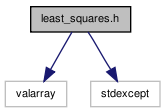# least_squares.h File Reference

`#include <valarray>`
`#include <stdexcept>`
Include dependency graph for least_squares.h:Go to the source code of this file.

## Namespaces

namespace  least_squares

## Defines

#define V(T)   std::valarray< T >
#define M(T)   std::valarray< V(T) >

## Typedefs

typedef Vx::value_type least_squares::T

## Functions

template<class T >
least_squares::V (T) linearFit(const M(T)&x
Returns parameters 'a' via ordinary least squares such that y = x·a.
template<class Vx , class Vy >
least_squares::V (typename Vx::value_type) polyFit(size_t degree
Returns fit 'a' of degree powers of x: y = [ 1 x .. xⁿ ] · a.
template<class T >
void least_squares::invert (M(T)&m)
Invert a matrix, in-place, using Gauss-Jordan elimination.
template void least_squares::invert (M(float)&m)
template void least_squares::invert (M(double)&m)
template least_squares::V (float) linearFit(const M(float)&x
template least_squares::V (double) linearFit(const M(double)&x
const Vx const Vy &y least_squares::if (degree >0)
least_squares::if (y.size()< degree)
least_squares::M (T) xn(V(T)(1
return least_squares::linearFit (xn, V(T)(&y, N))

## Variables

const Vx & least_squares::x
const Vx const Vy & least_squares::y
const size_t least_squares::N = y.size()
least_squares::degree

## Define Documentation

 #define M ( T ) std::valarray< V(T) >

Definition at line 62 of file least_squares.h.

 #define V ( T ) std::valarray< T >

Definition at line 61 of file least_squares.h.

 Tekkotsu v5.1CVS Generated Mon May 9 04:58:58 2016 by Doxygen 1.6.3